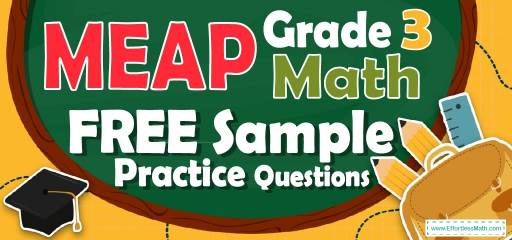# 3rd Grade MEAP Math FREE Sample Practice QuestionsPreparing your student for the 3rd Grade MEAP Math test? To succeed on the MEAP Math test, students need to practice as many real MEAP Math questions as possible.  There’s nothing like working on MEAP Math sample questions to measure your student’s exam readiness and put him/her more at ease when taking the MEAP Math test. The sample math questions you’ll find here are brief samples designed to give students the insights they need to be as prepared as possible for their MEAP Math test.

Check out our sample MEAP Math practice questions to find out what areas your student needs to practice more before taking the MEAP Math test!

Start preparing your student for the 2022 MEAP Math test with our free sample practice questions. Also, make sure to follow some of the related links at the bottom of this post to get a better idea of what kind of mathematics questions students need to practice.

## 10 Sample 3rd Grade MEAP Math Practice Questions

1- Which number correctly completes the subtraction sentence
$$8000 – 658 =$$_____ ?

A. 7,342

B. 7,452

C. 742

D. 7,458

2- Jason packs 14 boxes with flashcards. Each box holds 40 flashcards. How many flashcards Jason can pack into these boxes?

A. 56

B. 480

C. 540

D. 560

3- Which of the following statements describes the number 24,589?

A. The sum of two thousand, 4 thousand, five hundred, eighty tens, and nine ones

B. The sum of forty thousand, 2 thousand, five hundred, eight tens, and nine ones

C. The sum of twenty thousand, 4 thousand, fifty hundred, eighty tens, and nine ones

D. The sum of twenty thousand, 4 thousand, five hundred, eight tens, and nine ones

4- What is the value of “A” in the following equation?
$$21 + A + 9 = 44$$

A. 10

B. 12

C. 14

D. 20

5- Mr. smith usually eats four meals a day. How many meals does he eat in a week?

A. 21

B. 24

C. 28

D. 30

6- What is the value of $$A$$ in the equation $$72 ÷ A = 8$$

A. 2

B. 6

C. 7

D. 9

7- Use the models below to answer the question.
$$\img{https://appmanager.effortlessmath.com/public/images/questions/taar322d.JPG}$$
Which statement about the models is true?

A. Each shows the same fraction because they are the same size.

B. Each shows a different fraction because they are different shapes.

C. Each shows the same fraction because they both have 3 sections shaded.

D. Each shows a different fraction because they both have 3 shaded sections but a different number of total sections.

8- To what number is the arrow pointing?
$$\img{https://appmanager.effortlessmath.com/public/images/questions/taar3228.JPG}$$

A. 36

B. 38

C. 40

D. 42

9- Emily has 108 stickers and she wants to give them to nine of her closest friends. If she gives them all an equal number of stickers, how many stickers will each of Emily’s friends receive?___________

10- The following models are the same size and each is divided into equal parts.
The models can be used to write two fractions.
$$\img{https://appmanager.effortlessmath.com/public/images/questions/taar330.JPG}$$
Based on the models, which of the following statements is true?

A. $$\frac{2}{8}$$ is bigger than $$\frac{4}{16}$$.

B. $$\frac{2}{8}$$ is smaller than $$\frac{4}{16}$$.

C. $$\frac{2}{8}$$ is equal to $$\frac{4}{16}$$.

D. We cannot compare these two fractions only by using the models.

## Best 3rd Grade MEAP Math Prep Resource for 2022

1- A
$$8000 – 658 = 7342$$

2- D
$$14 × 40 = 560$$

3- C
24,589 is the sum of $$20,000; 4,000; 500; 80;$$ and 9

4- C
$$A = 44 – (21 + 9)$$
$$A = 14$$

5- C
In 1 day 4 meals so $$4 × 7 = 28$$ meals a week.

6- D
$$A = 72 ÷ 8$$
$$A= 9$$

7- D
the model for the first fraction is divided into 6 equal parts. We shade 3_6 to show the same amount as 1_2. The model for the second fraction is divided into 8 equal parts. We shade 3_ 8 that it shows these two models are different fractions.

8- C
The arrow shows exactly the middle of two numbers 35 and 45, so the answer is 40

9- 12
$$108 ÷ 9 = 12$$

10- C
the model for the first fraction is divided into 8 equal parts. We shade 2_ 8 to show the same amount as 1_ 4. The model for the second fraction is divided into 16 equal parts. We shade 4_ 16 to show the same amount as 1_ 4.